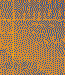## Randomly varying color of anagrams

##### Randomly varying color of anagrams
 Author Message nncheekGroup: Forum Members Posts: 8, Visits: 31 +xDave - Thursday, December 01, 2016+xDave - Thursday, December 01, 2016+xnncheek - Thursday, December 01, 2016 So, I added three shapes and tried to make it so that the quiz shows three squares as possible options (one for the correct color background on the anagram trial, and two distractor/wrong answer colors), each of which should be a different color, with only one color being correct. I used the code you had provided, with new shapes and this new list for the second shape color (probably done incorrectly), and then I edited the quiz trial as shown below, but now when I run it I only see one black box in the middle (50, 60) on the first quiz trial and then no boxes on the second quiz trial. I also get this error message: Failed to set the value of property 'color' on element 'shape.correctcolor'. Can you help me figure out what’s wrong here? I’m also worried that the shapes will always appear in the same place (i.e., the correct answer will always be in the same place), but ideally they’d be randomly positioned, though I’m not sure how to do that. / items = (1,2,3) / replace = true / not = (list.distractor_color.nextvalue;list.record_displaycolor.nextvalue) / stimulusframes = [1=recall_anagram, correctcolor, distractorcolor, distractor2_color, quit] / ontrialbegin = [shape.correctcolor.color = list.record_displaycolor.nextvalue; shape.distractorcolor.color=list.distractor_color.nextvalue;             shape.distractor2_color.color=list.distractor2_color.nextvalue; quit             ] / inputdevice = mouse / validresponse = (correctcolor, distractorcolor, distractor2_color) / correctresponse = (correctcolor) /shape=rectangle / size = (15%, 15%) / color = black /position = (20%, 60%) /shape=rectangle / size = (15%, 15%) / color = black /position = (50%, 60%) /shape=rectangle / size = (15%, 15%) / color = black /position = (80%, 60%) What you do is essentially this (essential changes from the example using elements in bold):/ blocks = [1=anagramblock; 2=quizblock]/ trials = [1-3 = anagramtrial]/ trials = [1-3 = quiztrial]//set the anagram text's color to a random value sampled from a list//store anagram item number & associated display color in empty lists//at runtime/ ontrialbegin = [text.anagram.textcolor = list.random_anagramcolor.nextvalue;]/ ontrialend = [list.record_anagram_item.appenditem(text.anagram.currentindex);    list.record_displaycolor.appenditem(list.random_anagramcolor.currentindex);    ]/ stimulusframes = [1=anagram]/ position = (50%, 60%)//random colors for the anagram text/ items = (red, green, blue)/ items = anagramitems/ 1 = "Anagram A"/ 2 = "Anagram B"/ 3 = "Anagram C"//lists to store the anagram item number//and associated randomly selected display color//those will be used for selection in the quiz block/ selectionmode = values.anagram_item//select a distractor color in quiz trials//selection must *not* be same as in list.record_displaycolor/ items = (1,2,3)/ replace = false/ selectionrate = always/ not = (list.record_displaycolor.nextvalue)/ ontrialbegin = [values.anagram_item = list.record_anagram_item.nextvalue;    shape.correctcolor.color = list.colors.item(list.record_displaycolor.nextvalue);    shape.distractorcolor.color = list.colors.item(list.distractor_color.nextvalue);    shape.distractorcolor2.color = list.colors.item(list.distractor_color.nextvalue);]/ ontrialend = [    list.distractor_color.reset();]/ stimulusframes = [1=recall_anagram, correctcolor, distractorcolor, distractorcolor2]/ inputdevice = mouse/ validresponse = (correctcolor, distractorcolor, distractorcolor2)/ correctresponse = (correctcolor)/ items = anagramitems/ select = values.anagram_item/ shape = rectangle/ size = (100px, 100px)/ vposition = 60%/ hposition = list.random_hposition.nextvalue/ shape = rectangle/ size = (100px, 100px)/ vposition = 60%/ hposition = list.random_hposition.nextvalue/ shape = rectangle/ size = (100px, 100px)/ vposition = 60%/ hposition = list.random_hposition.nextvalue/ items = (red, green, blue)/ items = (25%, 50%, 75%)/ selectionrate = always/ selectionmode = random/ replace = false/ anagram_item = 0Hold off a second; there's some stupid bug in the above code. Let me work out the kink and post an amended version ASAP.(The general approach is valid, though.)Here we go. Apologies for the confusion. For testing purposes -- so you can check that things work -- respond in the anagram trials with something like "a = red; " if Anagram A was displayed in the color red./ blocks = [1=anagramblock; 2=quizblock]/ trials = [1-3 = anagramtrial]/ trials = [1-3 = quiztrial]//set the anagram text's color to a random value sampled from a list//store anagram item number & associated display color in empty lists//at runtime/ ontrialbegin = [text.anagram.textcolor = list.random_anagramcolor.nextvalue;]/ ontrialend = [list.record_anagram_item.appenditem(text.anagram.currentindex);    list.record_displaycolor.appenditem(list.random_anagramcolor.currentindex);    ]/ ontrialend = [    values.responses = concat(values.responses, openended.anagramtrial.response);]/ stimulusframes = [1=anagram]/ position = (50%, 60%)//random colors for the anagram text/ items = (red, green, blue)/ items = anagramitems/ 1 = "Anagram A"/ 2 = "Anagram B"/ 3 = "Anagram C"//lists to store the anagram item number//and associated randomly selected display color//those will be used for selection in the quiz block/ selectionmode = list.record_anagram_item.currentindex//select a distractor color in quiz trials//selection must *not* be same as in list.record_displaycolor/ items = (1,2,3)/ replace = false/ selectionrate = always/ not = (list.record_displaycolor.nextvalue)/ ontrialbegin = [values.anagram_item = list.record_anagram_item.nextvalue;    shape.correctcolor.color = list.colors.item(list.record_displaycolor.nextvalue);    shape.distractorcolor.color = list.colors.item(list.distractor_color.nextvalue);    shape.distractorcolor2.color = list.colors.item(list.distractor_color.nextvalue);]/ ontrialbegin = [    values.correct_h = list.random_hposition.nextvalue;    values.dist1_h = list.random_hposition.nextvalue;    values.dist2_h = list.random_hposition.nextvalue;]/ ontrialend = [    list.distractor_color.reset();]/ stimulusframes = [1=recall_anagram, correctcolor, distractorcolor, distractorcolor2, debug]/ inputdevice = mouse/ validresponse = (correctcolor, distractorcolor, distractorcolor2)/ correctresponse = (correctcolor)/ items = ("<%values.responses%> Horizontal position of correct stimulus: <%values.correct_h%>")/ position = (50%, 10%)/ items = anagramitems/ select = values.anagram_item/ shape = rectangle/ size = (100px, 100px)/ vposition = 60%/ hposition = values.correct_h/ shape = rectangle/ size = (100px, 100px)/ vposition = 60%/ hposition = values.dist1_h/ shape = rectangle/ size = (100px, 100px)/ vposition = 60%/ hposition = values.dist2_h/ items = (red, green, blue)/ items = (25%, 50%, 75%)/ selectionrate = always/ selectionmode = random/ replace = false/ anagram_item = 0/ correct_h = 0%/ dist1_h = 0%/ dist2_h = 0%/ responses = ""/ separatefiles = trueThis seems to work perfectly--thanks so much!

#### Merge Selected

Merge into selected topic...

Merge into merge target...

Merge into a specific topic ID...